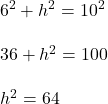## EL A square pyramid has a slant height of 10 ft, represented by s on the diagram. The length of a side of its base is 12 ft, and

Question

EL A square pyramid has a slant height of 10 ft,
represented by s on the diagram. The length of
a side of its base is 12 ft, and q is half of a side
of the base. What is the height of the pyramid?
Round to the nearest thousandth, if necessary.
(Hint: Use the Pythagorean theorem to find the height
of the pyramid)
S
(NOTE: image may not be shown to scale)

in progress 0
6 months 2021-09-01T10:33:57+00:00 1 Answers 0 views 0

8 ft

Step-by-step explanation:

Pythagorean theorem: the height of the pyramid, half of the base, and slant height make a right triangle

s = 10 ft

q = 6 ft

h = ?

s is the hypotenusetake the square root of 64:

h = 8